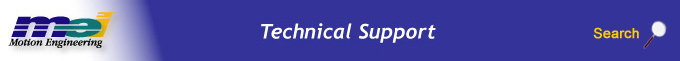.

### Using the Origin Variable

In servo control applications, the Command and Actual Positions for an Axis are generally used for position error calculations. On each sample, the controller reads the new Actual Positions from the feedback devices and calculates the new Command Positions.

To determine the relationship between Command and Actual Positions for an Axis and physical positions on the machine, you can use the Origin variable (which can be set by the host).

If it is important to set the Command or Actual Position to an exact value, you should use the mpiAxisOriginSet(...) function. Calling mpiAxisOriginSet(...) will modify both Command and Actual Positions by the same amount.

The Origin variable can be set in two different ways depending on the desired result. For example, assume the Command and Actual Positions are 10,000 and 10,024 counts respectively and that the axis is not moving.

Setting the Origin variable to 10,000 will result in Command Position = 0 and Actual Position = 24. Setting the Origin variable to 10,024 will result in Command Position = -24 and Actual Position = 0.

This second method is the most frequently used method for setting the new Origin after homing. Refer to the next table.

Position and Velocity criteria for generating motion completion events
 If Command Position = If Actual Position And you set Origin Variable Then Command Position = And Actual Position = 10,000 10,024 10,000 0 24 10,000 10,024 10,024 -24 0